Courses

# Test: Graphical Solution To Linear Equations

## 15 Questions MCQ Test Mathematics (Maths) Class 10 | Test: Graphical Solution To Linear Equations

Description
This mock test of Test: Graphical Solution To Linear Equations for Class 10 helps you for every Class 10 entrance exam. This contains 15 Multiple Choice Questions for Class 10 Test: Graphical Solution To Linear Equations (mcq) to study with solutions a complete question bank. The solved questions answers in this Test: Graphical Solution To Linear Equations quiz give you a good mix of easy questions and tough questions. Class 10 students definitely take this Test: Graphical Solution To Linear Equations exercise for a better result in the exam. You can find other Test: Graphical Solution To Linear Equations extra questions, long questions & short questions for Class 10 on EduRev as well by searching above.
QUESTION: 1

### Which of the following pair of linear equations is inconsistent?​

Solution:

Pair of linear equations are inconsistent when they are parallel. When the two equations are parallel ,we have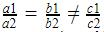.

In D, we have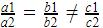which is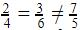, so it is inconsistent.

QUESTION: 2

### The pair of linear equations x + y + 10 = 0 and x + y – 7 = 0 has:

Solution:

We have a1, a2 the coefficients of x2,b1 and b2 coefficients of x and c1 and c2 the constant terms.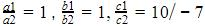So,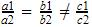a1a2=b1b2c1c2which is a case of parallel lines which which never meet. So there are no solutions obtainable for these equations.

QUESTION: 3

### The pair of linear equations 8x – 5y = 7 and 5x – 8y = -7 have :

Solution: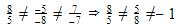So, they are intersecting lines. And intersecting lines meet at only one point. So only 1 solution is available.

QUESTION: 4

One equation of a pair of dependent linear equations is -5x + 7y = 2, the second equation can be :

Solution:

If a  system of two linear equation is consistent system and has an infinite number of solutions, it is dependent . When you graph the equations, both equations represent the same line.So we have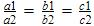which is satisfied by 10x – 14y = -4 only.

QUESTION: 5

Which of following is not a solution of 3a + b = 12?​

Solution:
QUESTION: 6

The number of solutions of the pair of linear equations x + 2y – 8 = 0 and 2x + 4y = 16 are:

Solution:

We have the equations x + 2y – 8 = 0 and 2x + 4y = 16 Where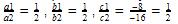Here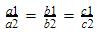which is the case of coincident lines . So there are infinitely many solutions.

QUESTION: 7

The pair of linear equations 3x + 2y = 5 and 2x – 3y = 7 will have

Solution:
QUESTION: 8

If the pair of equation has no solution, then the pair of equation is :

Solution:

The pair of equations has no solutions ,this means that the two equation does not have a common point which means that they does not meet each other anywhere.So the pair of lines which does not meet anywhere are parallel lines and parallel lines are inconsistent.

QUESTION: 9

Which of the following equation is not a linear equation?​

Solution:

Linear equation is an equation between two variables that gives a straight line when plotted on a graph. Linear equations have degree 1 only which means that power of the variables is 1 only. Since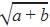does not have degree 1 its not a linear equation.

QUESTION: 10

A system of simultaneous linear equations has infinitely many solutions if two lines:

Solution:
QUESTION: 11

The pair of equations y = 0 and y = -7 has :

Solution:

y=0 is x-axis… since every point has y=0. y=-7 is a line parallel to x-axis passing through x=0,y=-7. So the two lines are parallel to each other and are inconsistent which means that it has no solutions because it will never meet.

QUESTION: 12

The following pair of linear equations 3x – 2y + 5 = 0, 5x + 7y = 2 will have

Solution:
QUESTION: 13

For what value of ‘K’ will the system of equations: 3x + y = 1, (2K – 1) x + (K – 1) y = 2K + 1 have no solution

Solution: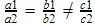is a case of parallel lines which never meet. So there are no solutions obtainable for these equations. So equations are inconsistent
3x + y = 1, (2K – 1) x + (K – 1) y = 2K + 1
b1=1,b2=k-1,c1=-1,c2=-2k-1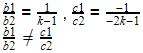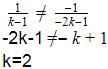QUESTION: 14

Which of the following pairs of equations represent inconsistent system?​

Solution: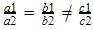is a case of parallel lines which never meet. So there are no solutions obtainable for these equations. So equations are inconsistent.
3x – y = -8 ,3x – y = 24
3x – y +8=0 ,3x – y -24=0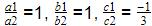So,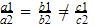Therefore the equations are inconsistent.

QUESTION: 15

Which of the following is not a solution of pair of equations 3x – 2y = 4 and 6x – 4y = 8?

Solution:

3x – 2y = 4 and 6x – 4y = 8
Putting x=5 and y=3
LHS=3(5)-2(3)
15-6=9
RHS=4
LHS ≠ RHS
Also,
6(5)-4(3)=30-12=18 ≠ 8